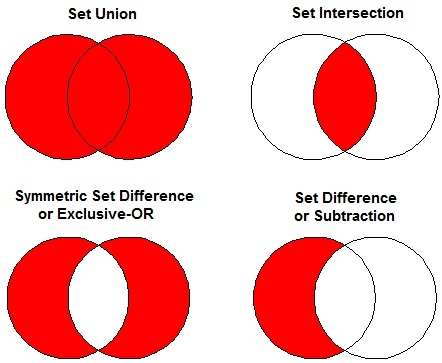# C++ Program to Implement Set_Difference in STL

C++Server Side ProgrammingProgramming

The difference of two sets is formed only by the elements that are present in the first set, not in the second set. The elements copied by the function come always from the first set in the same order. The elements in both the sets shall already be ordered.

Common set operations are −

• Set Union
• Set Intersection
• Symmetric Set Difference or Exclusive-OR
• Set Difference or Subtraction## Algorithm

Begin
Declare set vector v and iterator st.
Initialize st = set_difference (set1, set1 + n, set2, set2 +n, v.begin()))
Print the number of elements different between two sets.
End.

## Example Code

#include<iostream>
#include <algorithm>
#include <vector>
using namespace std;
int main () {
int set1[] = {5,6,7,8,9,10};
int set2[] = {1,2,3,4,6,7};
vector<int> v(10);
vector<int>::iterator it;
sort (set1, set1 + 6);
sort (set2, set2 + 6);
it = set_difference(set1, set1 + 6, set2, set2 + 6, v.begin());
v.resize(it - v.begin());
cout << "The difference between the sets has " << (v.size()) << " elements: "<<endl;
for (it = v.begin(); it != v.end(); ++it)
cout<< *it<<" ";
cout <<endl;
return 0;
}

## Output

The difference between the sets has 4 elements
5,8,9,10